# Practical exercise 4: ALIEN VS. EARTHLING

A comet orbits around the Sun, where its perihelion is at a distance of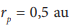and its aphelion is at a distance of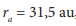see FIG. 2.

Specify:

a) main and minor axes, distance and numerical eccentricity of the ellipse,

b) the period of the comet,

c) how many times the comet in perihelion has a higher speed than in aphelion.Fig. 2 Schematic sketch of the comet's trajectory

Solution:

From the geometry of the ellipse we get: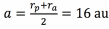,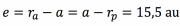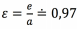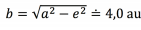. The comet's trajectory is expectedly very eccentric.

b) We write the 3rd Kepler's law in the form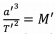where we substitute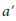with a numerical value in astronomical units, for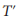ʹ a numerical value in years and for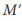multiples of the mass of the Sun. Because we are solving the orbit of a comet in the solar system,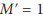. automatically applies. Thus,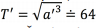applies as well. But as we know, in a given formulation of the law, all quantities are dimensionless. The numerical valuerepresents how many times the period is greater than the period of the Earth's rotation around the Sun (i.e. how many times the period is greater than one year). The period is thus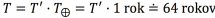.

It is possible to use another (but of course equivalent) notation of the 3rd Kepler's law. If we work in the SI system, we write the 3rd Kepler's law as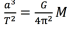We know the mass of the Sun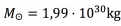, the gravitational constant has the value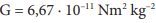and the semi

major axis is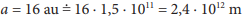.

From the 3rd Kepler's law we express the period: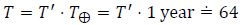years.

c) From the 2nd Kepler's law it follows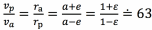.